# SSAT Elementary Level Math : How to find the whole from the part

## Example Questions

### Example Question #51 : Whole And Part

A movie theater can only sell as many movie tickets as there are seats in the theater. It sellstickets in advance,tickets online, andtickets at the box office. How many total seats does the theater have, assuming that the movie is a sell out?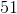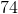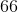Explanation:

To find the total number of seats in the theater, we need to find the total number of tickets sold. We do this by adding up the number of tickets sold in advance, online, and at the box office:in advance +online +at the box office =total seats

### Example Question #52 : Whole And Part

Mark's favorite cereal is now increasing the size of its product bypercent. Before, each box hadpieces. How many pieces does each box have now?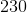pieces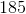pieces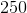pieces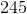pieces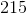piecespieces

Explanation:

First, find the value of the increase by takingof the original size,.of 200 is equal to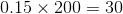Since this is the value of the increase, we can add it to the original size to find the new size.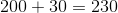### Example Question #10 : Partition Circles And Rectangles Into Two, Three, Or Four Equal Shares: Ccss.Math.Content.2.G.A.3

This square is split into __________.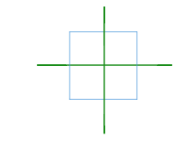fourths

thirds

halves

fourths

Explanation:

The square is split into four pieces, which means it is split into fourths.

### Example Question #1 : Compare Two Objects With A Measurable Attribute: Ccss.Math.Content.K.Md.A.2

Which shape is bigger?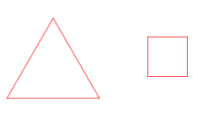Square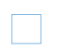They are they same size.

Triangle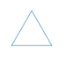TriangleExplanation:

The triangle is bigger than the square.

### Example Question #2 : Compare Two Objects With A Measurable Attribute: Ccss.Math.Content.K.Md.A.2

Which shape is bigger?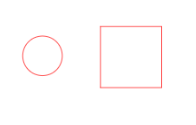The circle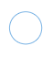They are the same size

The squareThe squareExplanation:

The square is bigger than the circle.

### Example Question #3 : Compare Two Objects With A Measurable Attribute: Ccss.Math.Content.K.Md.A.2

Which shape is bigger?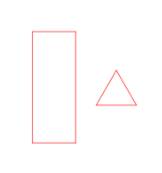They are the same size

The rectangle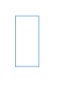The triangleThe rectangleExplanation:

The rectangle is bigger than the triangle.

### Example Question #4 : Compare Two Objects With A Measurable Attribute: Ccss.Math.Content.K.Md.A.2

Which shape is bigger?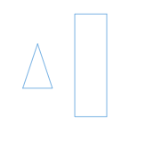The triangle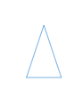They are the same size

The rectangleThe rectangleExplanation:

The rectangle is bigger than the triangle.

### Example Question #5 : Compare Two Objects With A Measurable Attribute: Ccss.Math.Content.K.Md.A.2

Which shape is bigger?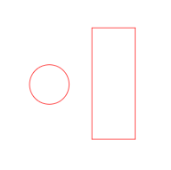The rectangleThe circleThey are the same size

The rectangleExplanation:

The rectangle is bigger than the circle.

### Example Question #6 : Compare Two Objects With A Measurable Attribute: Ccss.Math.Content.K.Md.A.2

Which shape is bigger?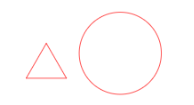The circleThey are the same size.

The triangleThe circleExplanation:

The circle is bigger than the triangle.

### Example Question #7 : Compare Two Objects With A Measurable Attribute: Ccss.Math.Content.K.Md.A.2

Which shape is bigger?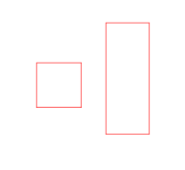They are the same size

The squareThe rectangle